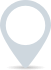# VAT calculator

Our VAT calculator can be used to determine the Value Added Tax (VAT) on a given amount. Helping individuals and businesses calculate the amount of VAT that should be added to or subtracted from a price.0%
£
.00

This calculator is intended for illustration purposes only and exact payment terms should be agreed with a lender before taking out a loan.

Gross price

£0

Tax amount

£0

## How to calculate VAT

To calculate Value Added Tax (VAT), you’ll need to follow these steps:

1. Determine the VAT Rate: First, find out the applicable VAT rate for the goods or services in question. In the UK, there are different rates for various items.

2. Calculate the VAT Amount: To calculate the VAT amount, multiply the original price by the VAT rate (expressed as a decimal). For example, if the original price is £100 and the VAT rate is 20%, the calculation would be:

VAT Amount = Original Price × VAT Rate
= £100 × 0.20
= £20

3. Total Price Including VAT: To find the total price including VAT, add the VAT amount to the original price:

Total Price = Original Price + VAT Amount
= £100 + £20
= £120

4. Total Price Excluding VAT: Conversely, if you have the total price including VAT and you want to find out the original price before VAT, you can use this formula:

Original Price = Total Price / (1 + VAT Rate)

Using the previous example:

Original Price = £120 / (1 + 0.20)
= £120 / 1.20
= £100

These calculations are essential for businesses and individuals to accurately handle VAT in transactions. Remember to always use the correct VAT rate for the specific goods or services you’re dealing with.

### Clever finance tips and the latest news

#### delivered to your inbox, every week